Previous Article in Journal
Living Radical Polymerization via Organic Superbase Catalysis
Previous Article in Special Issue
RC Beams Strengthened with Mechanically Fastened Composites: Experimental Results and Numerical Modeling
Open AccessArticle

# Behavior of FRP Bars-Reinforced Concrete Slabs under Temperature and Sustained Load Effects

byHizia Bellakehal 1,*,Ali Zaidi 1,*,Radhouane Masmoudi 2 andMohamed Bouhicha 1
1
Structure Rehabilitation and Materials Laboratory (SREML), University of Laghouat, Laghouat 03000, Algeria
2
Department of Civil Engineering, University of Sherbrooke, 2500, blvd Université, Sherbrooke, QC., J1K2R1, Canada
*
Authors to whom correspondence should be addressed.
Polymers 2014, 6(3), 873-889; https://doi.org/10.3390/polym6030873
Received: 28 November 2013 / Revised: 10 March 2014 / Accepted: 12 March 2014 / Published: 18 March 2014
(This article belongs to the Special Issue Selected Papers from "SMAR 2013")

## Abstract

The large temperature variation has a harmful effect on concrete structures reinforced with fiber reinforced polymer (FRP) bars. This is due to the significant difference between transverse coefficient of thermal expansion of these bars and that of the hardened concrete. This difference generates a radial pressure at the FRP bar/concrete interface, and may cause splitting cracks within concrete. This paper presents results of an experimental and analytical study carried out on FRP-reinforced concrete slabs subjected, simultaneously, to thermal and mechanical loads. The analytical model based on the theory of linear elasticity consists to evaluate combined effects of thermal and mechanical loads on the transverse expansion of FRP bars. Parameters studied in this investigation are the concrete cover thickness, FRP bar diameter, and the temperature variation. The thermal cycles were varied from −30 to +60 °C. Comparisons between analytical and experimental results show that transverse strains predicted from the proposed model are in good correlation with experimental results.
Keywords:

## 1. Introduction

The use of non-metallic fiber reinforced polymer (FRP) reinforcement as an alternative to steel reinforcement in concrete structures, particularly in hostile and aggressive environments, is gaining acceptance mainly due to its high corrosion resistance, and its high mechanical performance . However, the different mechanical behavior of non-metallic reinforcement, with respect to steel, involves some drawbacks namely the lack of thermal compatibility between concrete and FRP reinforcement. The coefficient of thermal expansion (CTE) in the transverse direction of FRP bars is typically much higher than its longitudinal CTE. For glass fiber reinforced polymer (GFRP) bars, whilst the longitudinal CTE (LCTE) is similar to that of concrete, the transverse CTE (TCTE) is three to eight times greater. Due to the difference between the transverse coefficients of thermal expansion of FRP bars and concrete, a radial pressure generated at the FRP bar/concrete interface induces tensile stresses within the concrete under the temperature increase. These tensile stresses may cause splitting cracks within concrete and eventually degradation of the member stiffness. As a consequence, important thermal strains take place just after the appearance of the first cracking concrete which occurs when the thermal stress in the concrete around GFRP bars, in different locations, reaches its tensile strength (fct). These thermal cracks may cause the degradation of the bond between GFRP bars and the surrounding concrete, and eventually, failure of the concrete cover if the confining action of concrete is not sufficient [2,3].
Although, temperature effects on mechanical properties of FRP are recognized by all documents reviewed, no guidance is given for the design of FRP reinforced concrete structures under temperatures variation effects . Hence, a better understanding of the thermal behavior of FRP reinforced concrete structures when subjected to large temperature variations is still required.
This paper presents experimental and analytical studies of the effective thermo-structural behavior of one-way GFRP-reinforced concrete slabs having different concrete cover thicknesses. Slabs were subjected simultaneously to service loads namely, a sustained mechanical load of 20% of the flexural ultimate load of slabs and a temperature variation from −30 to 60 °C, which represents, generally, the global temperature variation in many countries (North American, Arabian Gulf countries, etc.) Comparisons between experimental and analytical results prove that transverse thermal strains predicted from the proposed model are in good agreement with experimental results.

## 2. Experimental Section

#### 2.1. Description and Instrumentation of Slabs

A total of six series of concrete slab specimens reinforced with GFRP bars were fabricated for this experimental program, each series is constituted by three slabs: SA, SB, and SC. The slabs had dimensions of 500 mm wide, 195, 200, and 215 mm thick, 2500 mm total length, and 2000 mm span between supports (Table 1). Three series of slabs were reinforced longitudinally with six V-Rod glass FRP bars (GFRP) of 15.9 mm of diameter (bar #5), and three others were reinforced longitudinally with four V-Rod glass FRP bars of 19.1 mm of diameter (bar #6). The concrete covers used in this study (25, 30, and 45 mm) were selected according to the recommendations of ISIS-N°3-2007 . The slab SA was subjected to both: temperature variations (from −30 to +60 °C using the thermal room shown in Figure 1) and mechanical loads equal approximately to 20% of the theoretical ultimate load (Fu), as shown in Figure 2. The slab SB was subjected to a temperature variation from −30 to +60 °C. The Slab SC is the control specimen and was stored under room temperature (23 ± 1 °C). The mechanical load applied on the slab was distributed on two transverse loading lines at the middle span of slabs. The distance between the two loading lines was 500 mm. Except the concrete cover thickness, diameter of bars, temperature variation, and mechanical loads, all other parameters were kept constant for all slab specimens such as the length and the width of slabs, the shear span, and the compressive strength of concrete.
Table 1. Mechanical properties of concrete used.
Table 1. Mechanical properties of concrete used.
SlabsConcrete cover, c (mm)Bar diameter db (mm)c/dbLongitudinal modulus of elasticity, EC (GPa)Compression strength (MPa)Tensile strength (MPa)
SA195.25.162515.91.5726.17 ± 0.433.83 ± 11.94 ± 0.04
SA200.30.163015.91.8824.80 ± 0.830.39 ± 22.58 ± 0.04
SA215.45.164515.92.8326.17 ± 0.433.83 ± 11.94 ± 0.04
SA195.25.192519.11.3126.53 ± 0.334.77 ± 0.72.77 ± 0.15
SA200.30.193019.11.5827.34 ± 0.736.93 ± 22.92 ± 0.25
SA215.45.194519.12.3627.34 ± 0.736.93 ± 22.92 ± 0.25
Note: SA200.30.16 means: Slab SA, with thickness of 200 mm, reinforced with GFRP bars No. 16, concrete cover thickness c = 30 mm. For each series, the slabs SC and SB have the same properties than the slabs SA.
Figure 1. Environmental room including the four point flexural setup, slabs SA and SB.
Figure 1. Environmental room including the four point flexural setup, slabs SA and SB.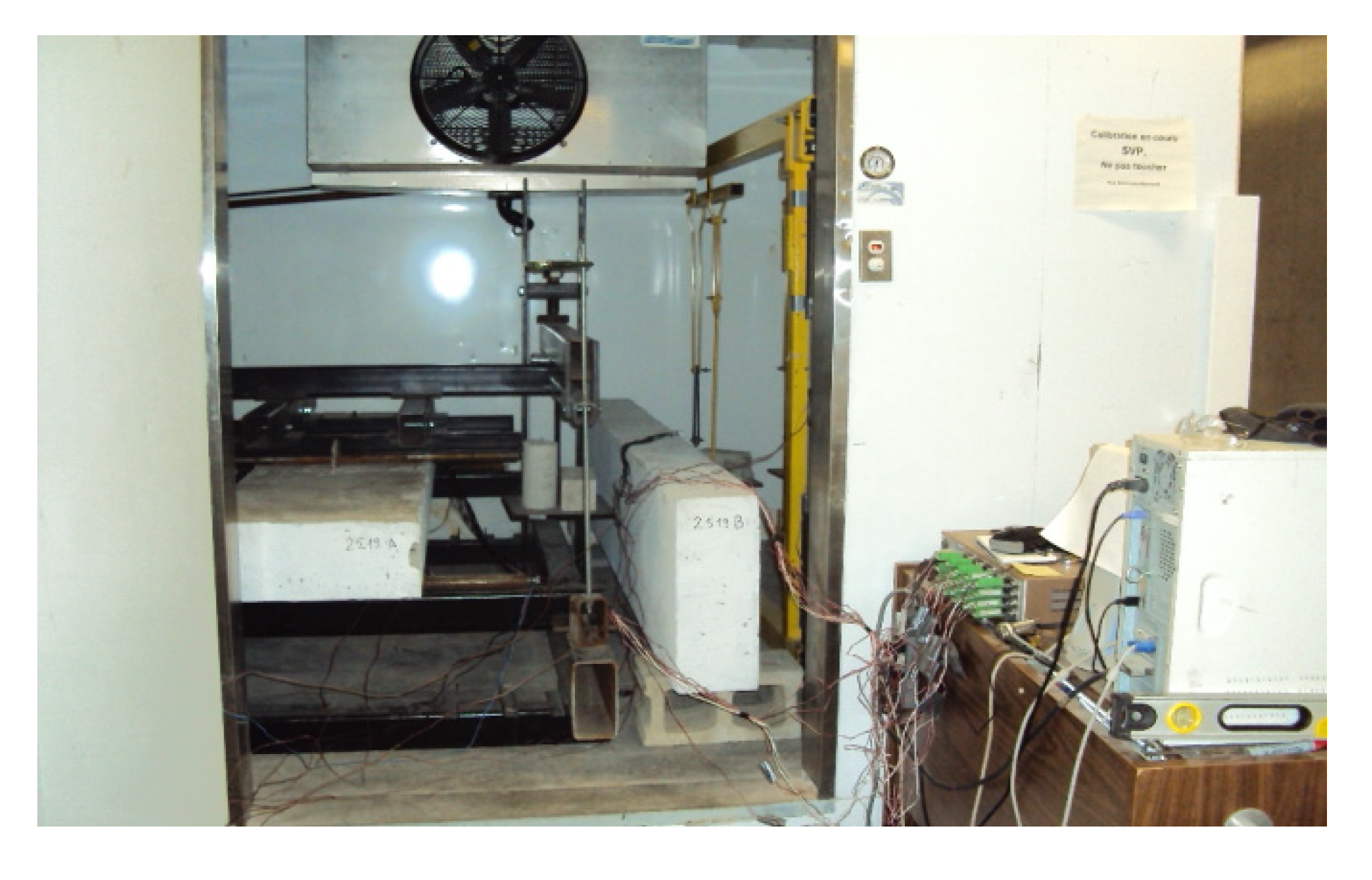Slabs were instrumented with strain gauges, thermocouples and LVDTs (linear variable differential transformers) in order to measure deformations, temperatures, and deflections, respectively. Each slab was instrumented by six strain gauges installed on three main bars in both transverse and longitudinal directions at mid-span of slabs. To measure the transverse and longitudinal strains of concrete, three strain gauges were placed on the lower tensioned surface of the slab during thermal tests. Then, three strain gauges were added on the upper compressed surface during the bending test. All strain gauges were installed on the mid-span. To measure the deflection and crack opening, two LVDTs and two sensor cracks were placed at mid-span and at 3/8 of the slab span. Each slab was instrumented by four thermocouples: two thermocouples are installed on two of the main bars, and tow thermocouples are installed on the upper and lower surface of slabs. Another one was attached inside the thermal room to measure the air temperature. All thermocouples were installed on the mid-span.

#### 2.2. Test Procedure

In the same conditions of slabs, six GFRP bars were tested (three bars N°16 and three N°19) under thermal and mechanical combined loads, each one was instrumented by two strain gages in the longitudinal and transverse directions of bar. The applied mechanical load is about 14% of the ultimate load of bars. This load representing the amount of axial load in GFRP bars of reinforcement of slabs SA (Figure 1 and Figure 3).
Figure 2. Typical Thermal cycles measured at FRP bar/concrete interface.
Figure 2. Typical Thermal cycles measured at FRP bar/concrete interface.Figure 3. Conditioned tensile test setup of GFRP bars.
Figure 3. Conditioned tensile test setup of GFRP bars.#### 2.3.1. Concrete

For each slab, six standard concrete cylinders of 150 mm × 300 mm were cast and cured with water for 28 days at the room temperature under the same conditions as the slab specimens. Cylinders were tested to evaluate the compression and tensile strengths of concrete at 28 days and immediately before slab specimen tests. The tensile strength was determined by the splitting test. The modulus of elasticity is calculated as recommended by the code CAN/CSA-S806-12 . Poisson’s ratio and coefficient of thermal expansion are assumed equal to νc = 0.17 and αc = 11.7 × 10−6/°C, respectively, as the concrete used is an ordinary concrete. The mechanical properties of concrete are presented in Table 1.

#### 2.3.2. Bars

The mechanical and thermal properties of GFRP bars used in this study are presented in Table 2. The modulus of elasticity is calculated as recommended by the code ACI 440.3R-04 . The average values of coefficients of thermal expansion of GFRP bars were measured by the Thermo Mechanical Analysis (TMA) test for temperatures variation from –30 °C to +60 °C. The other properties are the manufacturer’s specified values.
Table 2. Mechanical and thermal properties of GFRP reinforcing bars.
Table 2. Mechanical and thermal properties of GFRP reinforcing bars.
Bars diameter db (mm)15.919.1
Longitudinal Modulus of elasticity, Efl (GPa)47.0 ± 0.352.2 ± 1.2
Transverse modulus of elasticity, Eft (GPa)7.757.87
Poisson’s ratio in the longitudinal direction, νlt0.28 ± 0.0050.28 ± 0.008
Poisson's ratio in the transverse direction, νtt0.380.38
Ultimate tensile strength (MPa)700 ± 24691 ± 7
Guarantee tensile strength (MPa)683656
Ultimate tensile strain (%)1.50 ± 0.061.33 ± 0.03
TCTE* (αft) [×10−6 ]/°C27.35 ± 0.3522.45 ± 0.31
LCTE‡ (αfl) [×10−6]/°C6.81 ± 0.96.61 ± 0.1
Notes: * TCTE: Transverse Coefficient of Thermal Expansion; ‡ LCTE: Longitudinal Coefficient of Thermal Expansion.

#### 2.4.1. Thermo-Mechanical Behavior of FRP Bars

Figure 4 and Figure 5 show a comparison between slabs SA and SB in terms of longitudinal strains at the FRP bar/concrete interface as a function of temperatures (for a temperature variation from −30 to +60 °C and an applied mechanical load of 20% Fu), corresponding to FRP bar diameter N°16 and N°19, respectively.
Figure 4. Longitudinal strains of GFRP bars, db = 16 mm.
Figure 4. Longitudinal strains of GFRP bars, db = 16 mm.Figure 5. Longitudinal strains of GFRP bars, db = 19 mm.
Figure 5. Longitudinal strains of GFRP bars, db = 19 mm.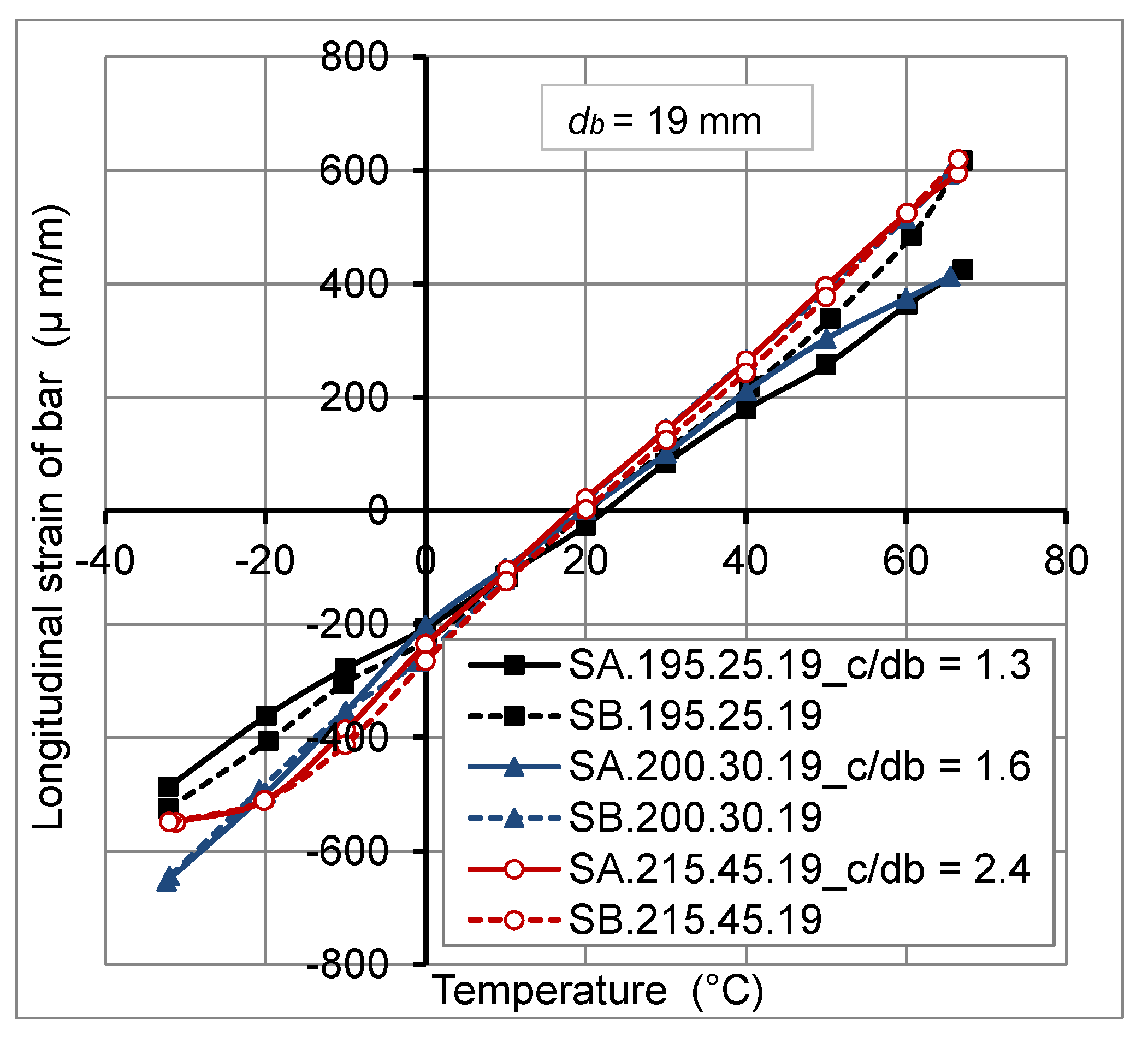Figure 6 and Figure 7 show a comparison between slabs SA and SB in terms of transverse strains of GFRP bars under the effect of thermal and mechanical loadings, corresponding to FRP bar diameter N°16 and N°19, respectively. From these figures, it can be observed that the mechanical load has no big influence on the transverse strains of GFRP bars.
Figure 8 shows that the variation of longitudinal and transverse thermal strain measuring at the interface between GFRP bars and concrete of the slab SA.200.30.16 in the cooling cycle from +20 to −30 °C coincide with those in the heating cycle from −30 to +20 °C. This proves that the thermal behavior of GFRP bars embedded in the concrete of actual slabs is linear elastic.
Figure 9 compares experimental results of slabs SA having different concrete cover thickness (25, 30, and 45 mm) and different GFRP bar diameter (N°16 and N°19), and the experimental results obtained from isolated GFRP bars (N°16 and N°19) tested under the same conditions of the tested slabs. This figure shows that the variation of concrete cover thickness did not affect transverse thermal strains of FRP bars. However, the increase of FRP bar diameter conducts to the decrease of these strains. This is due to the lower value of the transverse coefficient of thermal expansion of GFRP bars N°19 compared to that of GFRP bars N°16 (Table 2). It should be noted that the transverse expansion of FRP bars is governed by the resin, while the longitudinal expansion is governed by fibers . This is proved also by the experimental results obtained from isolated GFRP bars (N°16 and N°19) tested under the same conditions of the tested slabs (Figure 3 and Figure 9).
Figure 6. Transverse strains of GFRP bars, db = 16 mm.
Figure 6. Transverse strains of GFRP bars, db = 16 mm.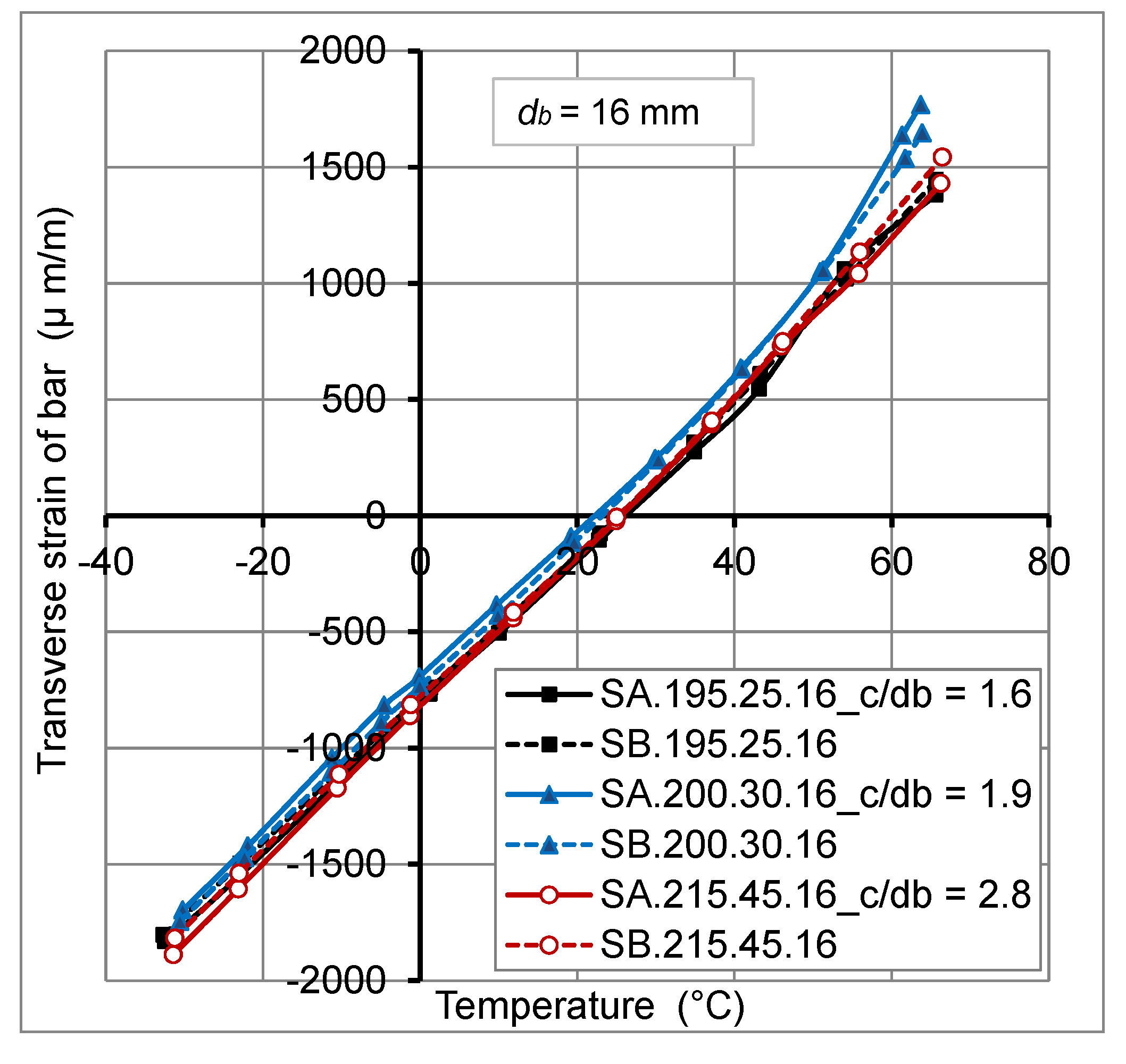Figure 7. Transverse strains of GFRP bars, db = 19 mm.
Figure 7. Transverse strains of GFRP bars, db = 19 mm.Figure 8. Behavior of GFRP imbedded in concrete of the slab SA.200.30.16undercooling-heating cycle and sustained load.
Figure 8. Behavior of GFRP imbedded in concrete of the slab SA.200.30.16undercooling-heating cycle and sustained load.Figure 9. Experimental transverse strains of GFRP bars for concrete slabs SA having different concrete cover and FRP bars diameter.
Figure 9. Experimental transverse strains of GFRP bars for concrete slabs SA having different concrete cover and FRP bars diameter.#### 2.4.2. Thermo-Mechanical Behavior of Concrete

Figure 10 and Figure 11 illustrate a comparison between slabs SA (subjected to combined thermal and mechanical loads) and SB (subjected to the thermal load only) in terms of transverse tensile concrete strains at external surface of concrete cover as a function of temperatures (for a temperature variation from −30 to +60 °C and an applied mechanical load of 20% Fu), corresponding to FRP bar diameter N°16 and N°19, respectively.
Figure 10. Transverse tensile concrete strains versus temperatures, db = 16 mm.
Figure 10. Transverse tensile concrete strains versus temperatures, db = 16 mm.Figure 11. Transverse tensile concrete strains versus temperatures, db = 19 mm.
Figure 11. Transverse tensile concrete strains versus temperatures, db = 19 mm.At the high temperature (60 °C), these Figures show that the thermal strains of slabs SA are generally lower than those of slabs SB. The difference is estimated by 5% to 15%. This is due to the decrease of the radial pressure and consequently the reduction of radial crack propagations through concrete cover from FRP bar/concrete interface for slabs SA, as explained in the Section 2.4.1. The results of slabs SA.200.30.19 and SA.215.45.19 cannot be considered, because the strain gauges were affected by the cracking concrete due to the mechanical load. For the low temperature, −30 °C), the effect of the mechanical load of 20% Fu, on transverse tensile concrete thermal strains, is insignificant.
Figure 12 exhibits a comparison between slabs SA (subjected to combined thermal and mechanical loads) and SB (subjected to the thermal load only) in terms of longitudinal tensile concrete strains as a function of temperature (for a temperature variation from −30 to +60 °C and an applied mechanical load of 20% Fu). Longitudinal thermal strains were measured only for the series of slabs having c/db equal to 1.6 and 1.9 with a bar diameter of 16 mm. It can be seen that the longitudinal thermal strains were not affected by the applied mechanical load.
Figure 12. Longitudinal tensile concrete strains versus temperatures, db = 16 mm.
Figure 12. Longitudinal tensile concrete strains versus temperatures, db = 16 mm.It should be noted that no thermal cracks have been observed at the outer surface of concrete cover at the end of experimental tests carried out on slabs under combined thermal and mechanical loads. Therefore, it can be concluded that ratios of concrete cover thickness to FRP bar diameter (c/db) used in this study (varied from 1.3 to 2.8) are sufficient to avoid failure of the concrete cover of GFRP—reinforced concrete slabs under combined thermal and mechanical service loads for materials used in this study. Also, it is noted that the tested slabs have been subjected, after thermal tests, to the four-points bending tests. The bending test results were published by Bellakehal et al. (2013) . It has been found that the shear ultimate strength and bond strength of GFRP bars of the tested slabs have not been affected by thermal and mechanical loads applied before four-points bending tests . So, we can conclude that the GFRP bars can be used as the principal reinforcement for concrete slabs subjected to the large variation of temperature (−30 °C to +60 °C). The temperature effect has been a most difficult drawback of FRP-reinforced concrete members, but there are no studies that investigate these members under large temperature variation simultaneously with mechanical load, as the manner described in this study.

## 3. Analytical Model

The analytical model is established to analyze the combined effect of thermal and mechanical loads on the behavior of a concrete cylinder concentrically reinforced with FRP bar. The model studied is based on the following assumptions:
-
A perfect bond between concrete and FRP bar.
-
The behavior of concrete and FRP bars is linear elastic.
-
The cross section of the concrete cylinder remains plane after deformation.
-
Absence of transverse reinforcing bars to evaluate only the contribution of the concrete cover to support the tensile stresses due to applied loads.
To determine thermal strains and stresses due to radial pressure P exerted by FRP bar on concrete cover under temperature increase ΔT, Rahman et al.  developed an analytical model based on the theory of elasticity of Timoshenko  for a concrete cylinder axially reinforced with FRP bar and subjected only to thermal loads. The expression of the radial pressure is given by:Where Δa/a is the transverse differential thermal strain; Ec: modulus of elasticity of concrete; Eft: modulus of elasticity of FRP bar in the transverse direction; νc: poisson’s ratio of concrete; νtt: poisson’s ratio of FRP bar in the transverse direction; r = b/a = (2c + db)/db: ratio of concrete cylinder radius “b” to FRP bar radius “a”; c: concrete cover thickness; db: FRP bar diameter.
Masmoudi et al.  and Zaidi et al.  have developed an analytical model based on the same theory. The expression of the radial pressure is given by:Where αft is the transverse coefficient of thermal expansion of FRP bars, and αc is the coefficient of thermal expansion of concrete.
This model has been modified to take into account the axial force in FRP bar due to applied mechanical loads. The circumferential strains in FRP bar (εft) and in concrete (εct) at the interface of FRP bar/concrete= a) due to the radial pressure P, the temperature variation ΔT, and the mechanical load are given by the following equations (Figure 13):Where εfl and εcl are, respectively, the longitudinal strains in the FRP bar and in the tensile concrete due only to the mechanical loading. The radial pressure P generated by the expansion of the FRP bar at the interface of FRP bar/concrete is obtained from the compatibility equation of circumferential deformations (εft = εct). Equations (3) and (4) give:Where:.
The radial stress (σρ) and the circumferential stress (σt) in a concrete element situated at a radius (ρ) from the center of the concrete cylinder due to the radial pressure (P), are given by: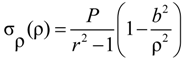The maximum circumferential stress at the concrete/bar interface (ρ = a) due to the radial pressure, obtained from Equation (7), is given by:Figure 13. Concrete cylinder concentrically reinforced with FRP bar.
Figure 13. Concrete cylinder concentrically reinforced with FRP bar.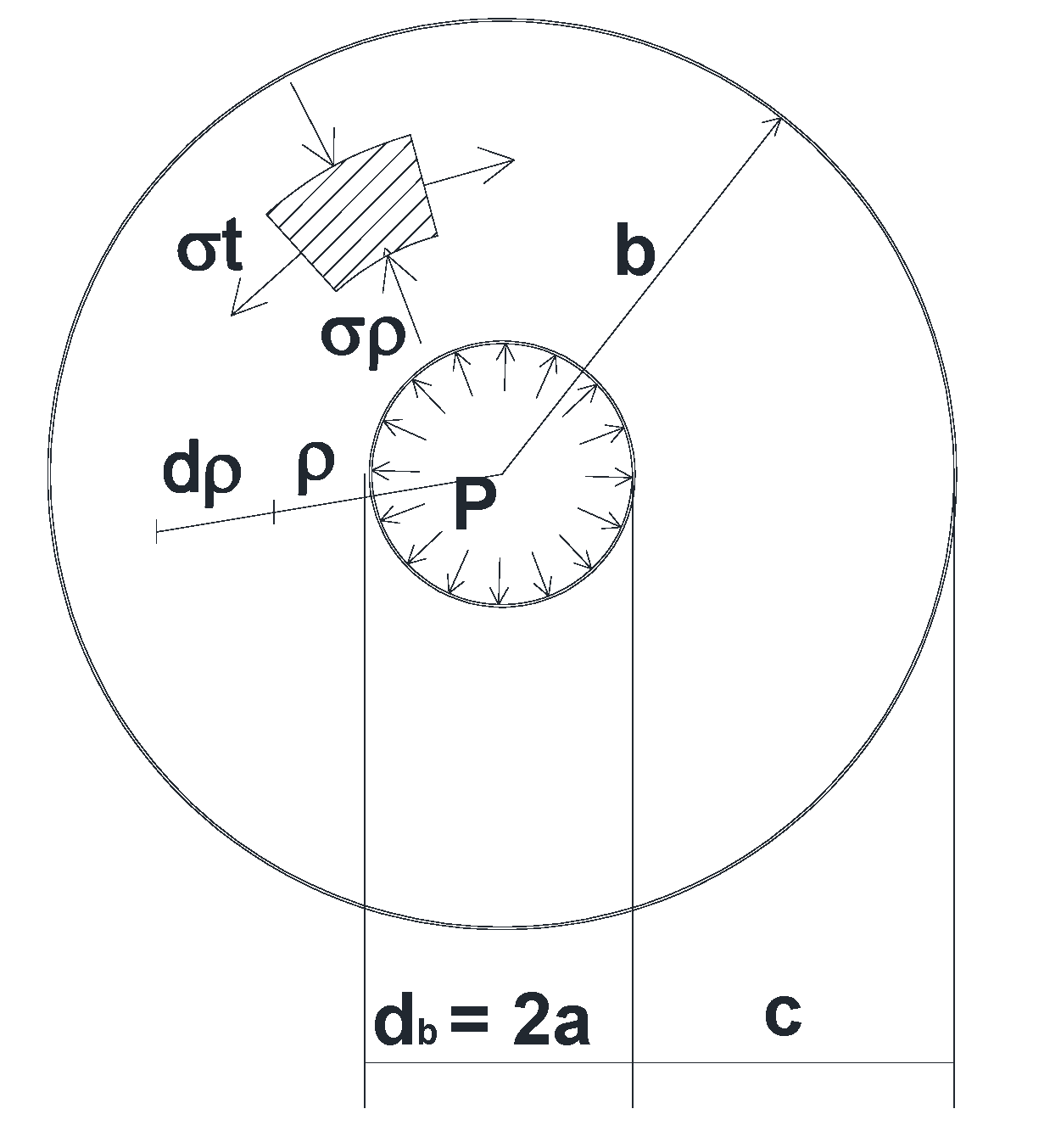## 4. Comparison of Analytical and Experimental Results

Figure 14 and Figure 15 present typical curves which compare experimental results with analytical predictions given by Equations (2) and (3), in terms of transverse thermal strains, at the FRP bar/concrete interface as a function of temperature variation (ΔT) at the mid-span of slabs SA. These slabs were tested under combined thermal and mechanical loads (with temperature variation from −30 to +60 °C and mechanical load of 20% Fu). It should be noted that the reference temperature 23 ± 1 °C.
It is observed that experimental results are widely higher than those obtained from the analytical model. This divergence is probably due to the development of circumferential cracks around FRP bars due to radial tensile stresses developed at low temperature added to those of the shrinkage effect. However, at high temperature, this divergence is due to radial cracks caused by the thermal expansion of FRP bars when the temperature increases. These cracks were not taken into account in the assumptions of the analytical model based on the theory of elasticity.
To determine transverse strains of FRP bars, the analytical model (Equation (3)) was modified to fit the experimental results obtained from FRP-reinforced concrete slabs tested under a temperature variation from −30 to + 60 °C applied simultaneously with a mechanical loading of 20% of the ultimate load of slabs. The proposed model is given by:Figure 14 and Figure 15 show that transverse strains predicted from the proposed model are in good agreement with experimental results. It should be noted that the proposed model is only valid for materials used in this study.
Figure 14. Transverse strain of FRP bars of slab SA.30.16—Experimental and analytical results comparison, c/db = 1.9.
Figure 14. Transverse strain of FRP bars of slab SA.30.16—Experimental and analytical results comparison, c/db = 1.9.Figure 15. Transverse strain of FRP bars of slab SA.45.16—Experimental and analytical results comparison, c/db = 2.8.
Figure 15. Transverse strain of FRP bars of slab SA.45.16—Experimental and analytical results comparison, c/db = 2.8.## 5. Conclusions

Eighteen large-scale bars FRP-reinforced concrete slabs were cast and tested. Slabs were divided in six series. Each one was constituted of three slabs. The first slab was subjected to both temperature variations and mechanical loads. The second slab was subjected only to temperature variation. The last one was the control slab and was stored under room temperature. The studied parameters used were concrete cover thickness, FRP bar diameter, and temperatures. The temperature was varied from −30 °C to +60 °C and the applied mechanical load was 20% of slab ultimate load (Fu). The main objective of this study is to investigate the mechanical load effect on the behavior of GFRP bars reinforced concrete slabs subjected to large temperature ranges (−30 °C to +60 °C). Although, temperature effects have been a most difficult drawback of FRP-reinforced concrete members, there are no studies which investigate these members under large temperature variation simultaneously with mechanical loads, as the manner described in this study. It should be noted that these results are valid for slabs with materials used in this study. Based on the analysis of the analytical and experimental results in terms of thermal strains of concrete and GFRP bars, the following conclusions can be drawn:
• The mechanical load effect of 20% of the ultimate load (Fu) of reinforced concrete slabs has no significant effect on the transverse thermal strains of GFRP bars embedded in concrete under temperature variation from −30°C to +60°C.
• At high temperature (>40°C), longitudinal thermal strains at the GFRP bar/concrete interface have been reduced under applied mechanical loads. This reduction reached 30%, for a temperature of +60°C, due to the decrease of radial pressure generated at the interface. However, for temperatures variation from −30°C to +40°C, the mechanical load has no big influence on longitudinal thermal strains at the GFRP bar/concrete interface.
• The thermo-mechanical behavior of GFRP bars embedded in concrete of actual slabs is linear elastic.
• The concrete cover thickness variation has no big effect on transverse thermal strains at GFRP bar/concrete interface, for temperature variation from −30 °C to +60 °C. However, the transverse thermal strains decrease with the increase of FRP bar diameter.
• At high temperature, transverse tensile concrete strains at external surface of concrete cover were reduced under mechanical load. This reduction varied from 5% to 15%, for a temperature of +60 °C, due to the decrease of the radial pressure and consequently the reduction of radial crack propagations through concrete cover. While, for the low temperature (−30 °C), the mechanical load of 20% Fu, has no remarkable effect on transverse tensile concrete strains.
• Ratiosof concrete cover thickness to FRP bar diameter (c/db) varied from 1.3 to 2.8 are sufficient to avoid failure of the concrete cover of GFRP bars—reinforced concrete slabs under combined thermal and mechanical service loads for materials used in this study.
• The transverse strains, at FRP bar/concrete interface of concrete slab under thermal and mechanical loads, predicted from the analytical model are greater than those obtained from experimental tests. This is due to the presence of cracks produced within concrete at the interface which is not considered in the linear analytical model based on the theory of elasticity.
• The transverse strains, at FRP bar/concrete interface, predicted from the proposed model, are in good agreement with experimental results obtained from GFRP bars-reinforced concrete slabs under the combined thermal load (from −30 °C to +60 °C) and mechanical load (20% of the ultimate load of slabs).

## Nomenclature

 c Concrete cover thickness db FRP bar diameter Ec Modulus of elasticity of concrete Eft Modulus of elasticity of FRP bar in the transverse direction fct Tensile strength Fu Ultimate load f’c28 Compressive concrete strength P Radial pressure r = b/a ratio of concrete cylinder radius “b” to FRP bar radius “a” αft Transverse coefficient of thermal expansion of FRP bars αc Coefficient of thermal expansion of concrete ΔT Temperature variation εft Circumferential strains in FRP bar at the interface of FRP bar/concrete εct Circumferential strains in concrete at the interface of FRP bar/concrete εfl Longitudinal strain in the FRP bar due only to the mechanical loading εcl Longitudinal strain in the tensile concrete due only to the mechanical loading νc Poisson’s ratio of concrete νtt Poisson’s ratio of FRP bar in the transverse direction νlt Poisson’s ratio of FRP bar (force applied in longitudinal direction and strains measured in transverse direction) ρ Radius from the center of the concrete cylinder σρ Radial stress in concrete cover σt Circumferential stress in concrete cover σt, max Maximum circumferential stress in concrete cover

## Acknowledgments

Great thanks to the personnel of the Civil Engineering Department of the Sherbrooke University (Canada) for their helps. Also, Special thanks to “Ministère de l’Enseignement Supérieur et de la Recherche Scientifique” of Algeria for the PhD scholarship granted to Bellakehal (PhD candidate) and to the manufacturer (Pultrall Inc., Québec, Canada) for providing FRP bars. The research reported in this paper carried out in the Sherbrooke University was partially sponsored by the Natural Sciences and Engineering Research Council of Canada (NSERC). The authors also acknowledge the contribution of the Canadian Foundation for Innovation (CFI) for the infrastructure used to conduct testing. The opinion and analysis presented in this paper are those of the authors.

## Author Contributions

The topic of this study was proposed by Ali Zaidi. The whole work was done by Hizia Bellakehal. The comments and revision were done by Ali Zaidi. Finally, it is noted that this work was supervised by Radhouane Masmoudi and Mohamed Bouhicha.

## Conflicts of Interest

The authors declare no conflict of interest.

## References

1. Pendhari, S.S.; Kant, T.; Desai, Y.M. Application of polymer composites in civil construction: A general review. Compos. Struct. 2008, 84, 114–124. [Google Scholar] [CrossRef]
2. Zaidi, A.; Masmoudi, R. Thermal effect on FRP-reinforced concrete slabs. In Proceedings of 1st International Structural Specialty Conference, CSCE, Alberta, Canada, 23–26 May 2006.
3. EL-Zaroug, O.; Forth, J.; Ye, J.; Beeby, A. Flexural performance of concrete slabs reinforced with GFRP and subjected to different thermal histories. In Proceedings of 8th International Symposium on Fiber Reinforced Polymer Reinforcement for Concrete Structures, FRPRCS-8, Patras, Greece, 16–18 July 2007.
4. Elbadry, M.; Elzaroug, O. Control of cracking due to temperature in structural concrete reinforced with CFRP bars. Compos. Struct. 2004, 64, 37–45. [Google Scholar] [CrossRef]
5. Intelligent Sensing for Innovative Structures (ISIS Canada). Design Manual N°3Reinforcing Concrete Structures with Fibre Reinforced Polymers; ISIS: Winnipeg, Manitoba, Canada, 2007. [Google Scholar]
6. Bellakehal, H.; Zaidi, A.; Masmoudi, R.; Bouhicha, M. Combined effect of sustained load and freeze-thaw cycles on one-way concrete slabs reinforced with glass fibre-reinforced polymer. Can. J. Civil Eng. 2013, 40, 1060–1067. [Google Scholar] [CrossRef]
7. Canadian Standards Association (CSA). Design and Construction of Building Structures with Fibre-Reinforced Polymers; CAN/CSA S806-12; CSA: Mississauga, Ontario, Canada, 2012. [Google Scholar]
8. American Concrete Institute (ACI). Guide Test Methods for Fibre-Reinforced Polymers (FRPs) for Reinforcing or Strengthening Concrete Structures; ACI 440; American Concrete Institute: Farmington Hills, MI, USA, 2004. [Google Scholar]
9. Vogel, H.; Svecova, D. Effect of temperature on concrete cover of FRP prestressed elements. In Proceeding of Advanced Composite Materials in Bridges and Structures (IV ACMBS), Alberta, Canada, 20–23 July 2004.
10. Rahman, H.A.; Kingsley, C.Y.; Taylor, D.A. Thermal stress in FRP reinforced concrete. In Proceeding of Annual Conference of the Canadian Society for Civil Engineering, Ottawa, Canada, 1–3 June 1995.
11. Timoshenko, S.P.; Goodier, J.N. Calcul des cylindres a parois épaisses et disques tournants. In Théorie d’élasticité; Librairie Polytechnique Ch. Béranger: Paris, France, 1961; pp. 514–521. [Google Scholar]
12. Masmoudi, R.; Zaidi, A.; Gérard, P. Transverse thermal expansion of FRP bars embedded in concrete. J. Compos. Constr. 2005, 9, 377–387. [Google Scholar] [CrossRef]
13. Zaidi, A.; Masmoudi, R. Thermal effect on fiber reinforced polymer reinforced concrete slabs. Can. J. Civil Eng. 2008, 35, 312–320. [Google Scholar] [CrossRef]